Arduino

# Machine Learning for Makers: How to Voice Control an RGB LEDJune 28, 2018 by Muhammad Aqib
Share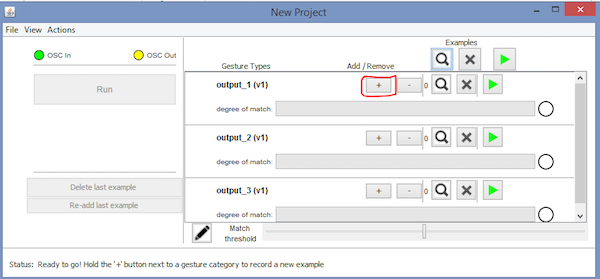Use the machine learning program Wekinator and an Arduino UNO to control the color of an RGB LED with your voice!

## Software

 1 Wekinator

## Hardware

 1 Arduino UNO 1 RGB LED 1 220-ohm resistor

In this article, which is part 3 of a larger machine learning series, you will learn how you can change the color of an RGB LED by just saying the color. We will use the Wekinator software as the machine learning platform, and after training Wekinator, we will use processing to get Wekinatorâ€™s output, from where we will send it to the Arduino.

Catch up on the rest of the Wekinator Machine Learning series here:

### Circuit Diagram

Connect the longest leg of the RGB LED to the ground of Arduino. Connect the other legs to pins 9, 10, and 11 of Arduino through the 220-ohm resistor as shown in the below circuit diagram.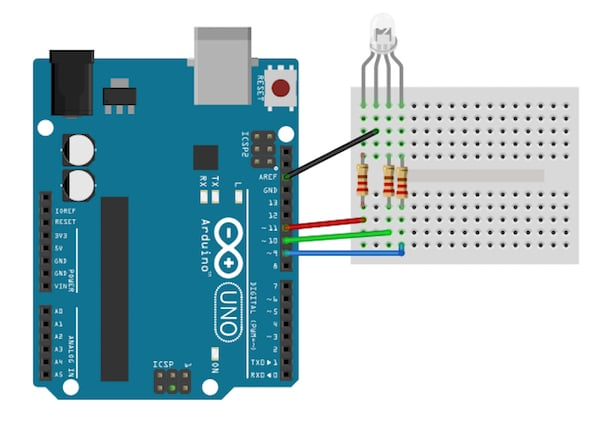### How to Run the Program

First of all, paste the code given for Arduino at the end of this post in the Arduino IDE and upload the code.

Then you will need to download the sketch from the examples page of Wekinator.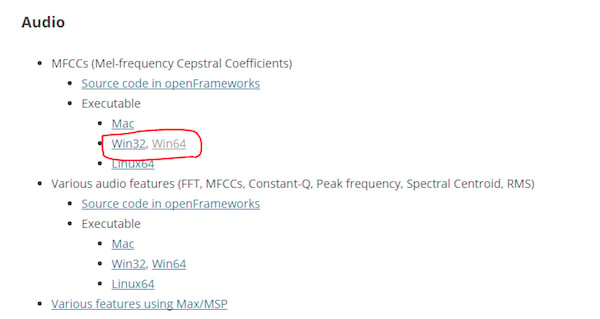After downloading, unzip it and run the â€œ.exeâ€ file. It will look like as shown below. Now you need a microphone to give the input to Wekinator. If you have attached an external microphone, make sure it is selected in the sound settings of your computer.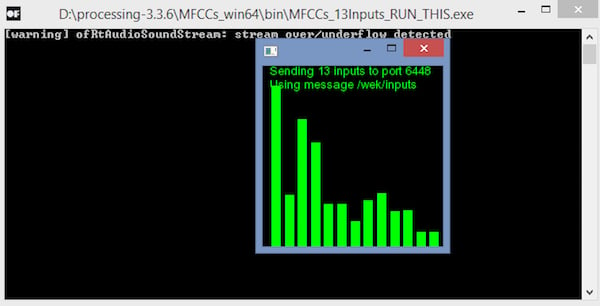You will require another sketch (â€œoutput sketchâ€) to get the output from Wekinator. This sketch is given at the end of this post. Paste it into the new processing window and run the sketch.

Now open the Wekinator and make the settings as shown in the figure below. Set the inputs to 13 and the outputs to 1. Set the type to â€œAll dynamic time warpingâ€ with 3 gesture types and click on â€œnextâ€.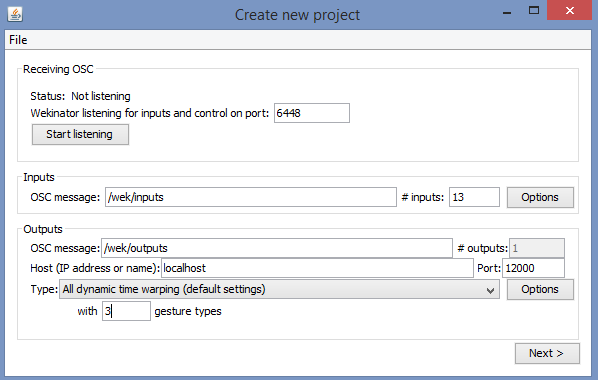Now hold the â€œ+â€ button in front of the output_1 and say â€œredâ€.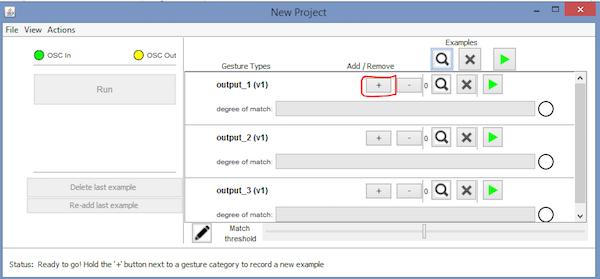Then hold the â€œ+â€ button in front of the output_2 and say â€œgreenâ€.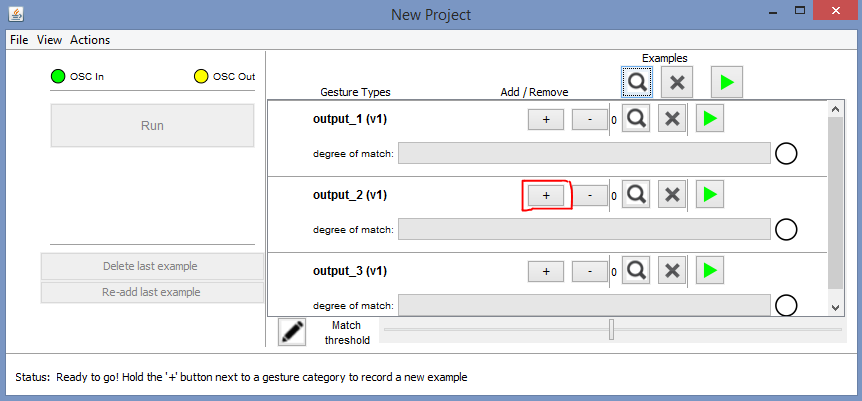Then hold the â€œ+â€ button in front of output_3 and say â€œblueâ€.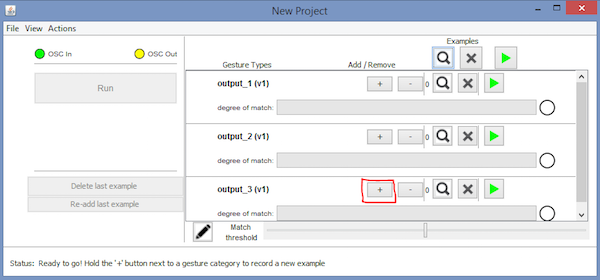After that, click on â€œTrainâ€, then click â€œRunâ€. Now the color of the RGB LED will change according to the color name you say.

### Arduino Code

``````#include <VSync.h>    //Including the library that will help us in receiving and sending the values from processing
Put the number of values to synchronize in the brackets */

/* The below variable will be synchronized in the processing
and they should be same on both sides. */
int output;

// Initializing the pins for led's
int red_light_pin= 11;
int green_light_pin = 10;
int blue_light_pin = 9;

void setup()
{
/* Starting the serial communication because we are communicating with the
Processing through serial. The baudrate should be same as on the processing side. */
Serial.begin(19200);
pinMode(red_light_pin, OUTPUT);
pinMode(green_light_pin, OUTPUT);
pinMode(blue_light_pin, OUTPUT);

// Synchronizing the variable with the processing. The variable must be int type.
}

void loop()
{
// Receiving the output from the processing.

// Matching the received output to light up the RGB LED
if (output == 1)
{
RGB_color(255, 0, 0); // Red
}
else if (output == 2)
{
RGB_color(0, 255, 0); // Green
}
else if (output ==3)
{
RGB_color(0, 0, 255); // Blue
}
}

void RGB_color(int red_light_value, int green_light_value, int blue_light_value)
{
analogWrite(red_light_pin, red_light_value);
analogWrite(green_light_pin, green_light_value);
analogWrite(blue_light_pin, blue_light_value);
}``````

### Processing Code (Output Sketch)

``````import vsync.*; // Importing the library that will help us in sending and receiving the values from the Arduino
import processing.serial.*;  // Importing the serial library

// Below libraries will connect and send, receive the values from wekinator
import oscP5.*;
import netP5.*;

// Creating the instances
OscP5 oscP5;
ValueSender sender;

// This variable will be syncronized with the Arduino and it should be same on the Arduino side.
public int output;

void setup()
{
// Starting the serial communication, the baudrate and the com port should be same as on the Arduino side.
Serial serial = new Serial(this, "COM10", 19200);
sender = new ValueSender(this, serial);

// Synchronizing the variable as on the Arduino side.
sender.observe("output");

// Starting the communication with wekinator. listen on port 12000, return messages on port 6448
oscP5 = new OscP5(this, 12000);
}

//This is called automatically when OSC message is received
void oscEvent(OscMessage theOscMessage) {
{
output = 1;
}
{
output = 2;
}
{
output = 3;
}
else
{

}
}

void draw()
{
//  Nothing to be drawn for this example
}``````